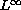Electron. J. Diff. Eqns., Vol. 1999(1999), No. 48, pp. 1-8.

### On the smallness of the (possible) singular set in space for 3D Navier-Stokes equations Zoran Grujic

Abstract:
We utilizeestimates on the complexified solutions of 3D Navier-Stokes equations via a plurisubharmonic measure type maximum principle to give a short proof of the fact that the Hausdorff dimension of the (possible) singular set in space is less or equal 1 assuming chaotic, Cantor set-like structure of the blow-up profile.

Submitted August 20, 1999. Published December 3, 1999.
Math Subject Classifications: 35Q30, 76D03.
Key Words: Navier-Stokes equations, singular set, turbulence.

Show me the PDF file (111K), TEX file, and other files for this article.

Zoran Grujic
Department of Mathematics
University of Texas
Austin, TX 78712, USA
e-mail: grujic@math.utexas.edu